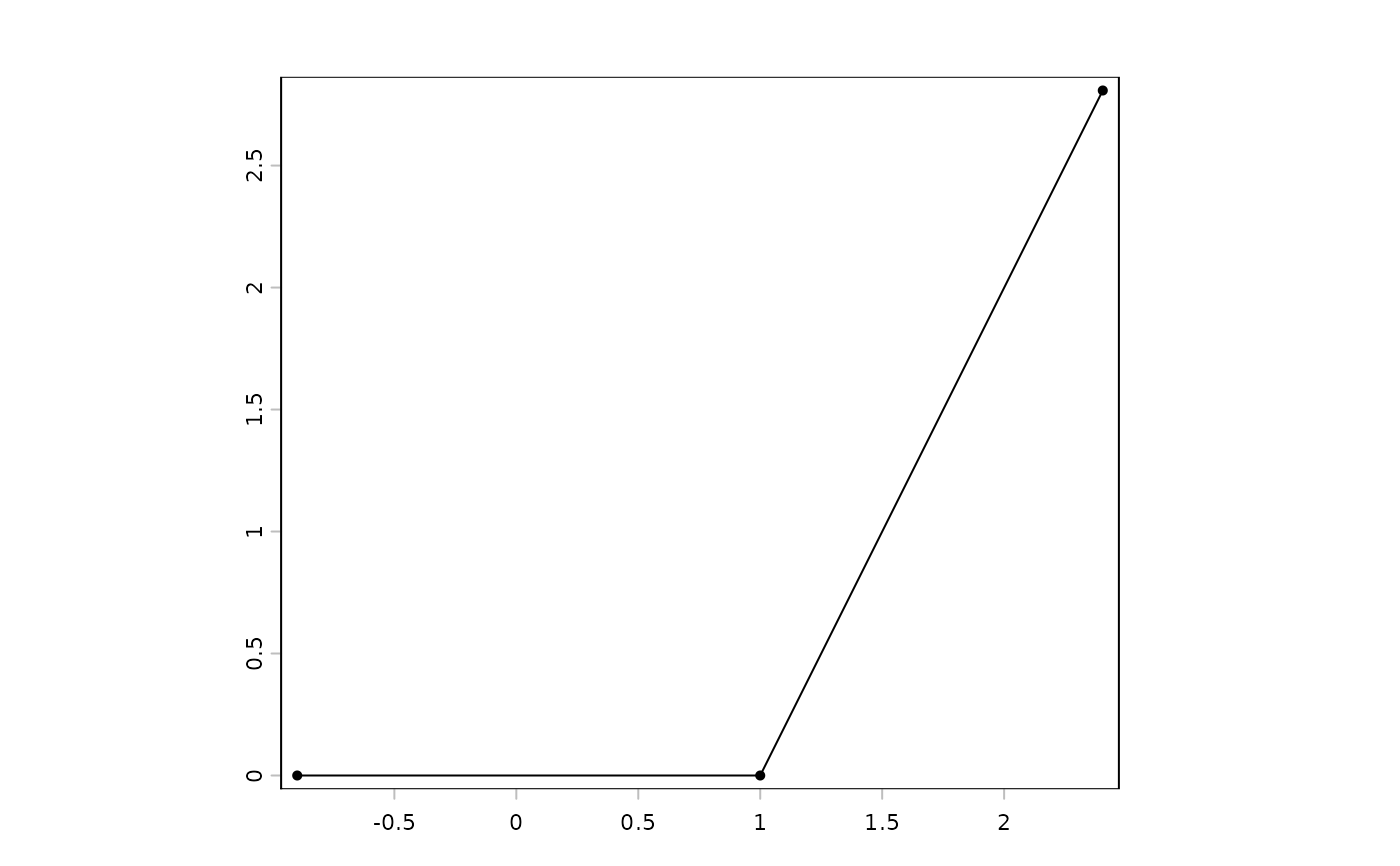Elongate SpatVector lines

# S4 method for SpatVector
elongate(x, length=1, flat=FALSE)

## Arguments

x

SpatVector

length

positive number indicating how much the lines should be elongated at each end. The unit is meter is the crs is lonlat and it is the same as the linear unit of the crs on other cases (also meter in most cases)

flat

logical. If TRUE, the earth's curvature is ignored for lonlat data, and the distance unit is degrees, not meter

## Value

SpatVector

buffer, crop and erase

## Examples

v <- vect(cbind(c(0,1,2), c(0,0,2)), "lines", crs="lonlat")
e <- elongate(v, 100000)
plot(e)
points(e)geom(e)
#>      geom part          x        y hole
#> [1,]    1    1 -0.8983153 0.000000    0
#> [2,]    1    1  1.0000000 0.000000    0
#> [3,]    1    1  2.4044661 2.807681    0